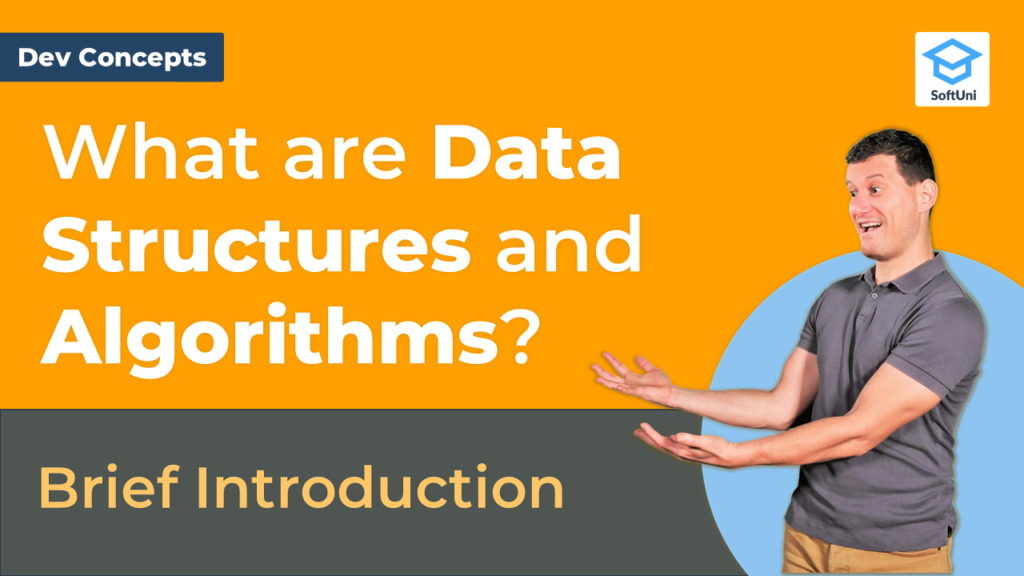# What are Data Structures and Algorithms? [Dev Concepts #5]

What are Data Structures? How Data Structures work with Algorithms? Learn this and more in this dev lesson!Do you know what data structures and algorithms are and how they work together? It’s important to learn data structures and algorithms properly so you can understand the organizing principles behind web development and programming work. Get familiar with the common categories of algorithms such as Search, Sorting, Graph/Tree traversing.

A data structure is how we organize data in a virtual system. We can think of this as tables of data. An algorithm is a sequence of steps executed by a machine or computer. Each algorithm interacts with different data using the same complexity scale. Data structures can be linear structures (such as arrays and lists), tree-like structures (such as balanced trees), graph-like structures (such as graphs), hash-based structures (such as hash-tables), and others.

## In this video we review the following topics:

• Data Structures
• List of Numbers Demo
• Trees and Traversal Algorithms